## 1.1 Problem Description

Netflix is all about connecting people to the movies they love. To help customers find those movies, they developed world-class movie recommendation system: CinematchSM. Its job is to predict whether someone will enjoy a movie based on how much they liked or disliked other movies. Netflix use those predictions to make personal movie recommendations based on each customer’s unique tastes. And while Cinematch is doing pretty well, it can always be made better.

Now there are a lot of interesting alternative approaches to how Cinematch works that netflix haven’t tried. Some are described in the literature, some aren’t. We’re curious whether any of these can beat Cinematch by making better predictions. Because, frankly, if there is a much better approach it could make a big difference to our customers and our business.

Credits: https://www.netflixprize.com/rules.html

## 1.2 Problem Statement

Netflix provided a lot of anonymous rating data, and a prediction accuracy bar that is 10% better than what Cinematch can do on the same training data set. (Accuracy is a measurement of how closely predicted ratings of movies match subsequent actual ratings.)

## 1.3 Sources

• https://www.netflixprize.com/rules.html
• https://www.kaggle.com/netflix-inc/netflix-prize-data
• Netflix blog: https://medium.com/netflix-techblog/netflix-recommendations-beyond-the-5-stars-part-1-55838468f429 (very nice blog)
• surprise library: http://surpriselib.com/ (we use many models from this library)
• surprise library doc: http://surprise.readthedocs.io/en/stable/getting_started.html (we use many models from this library)
• installing surprise: https://github.com/NicolasHug/Surprise#installation
• Research paper: http://courses.ischool.berkeley.edu/i290-dm/s11/SECURE/a1-koren.pdf (most of our work was inspired by this paper)

## 1.4 Real world/Business Objectives and constraints

Objectives:

1. Predict the rating that a user would give to a movie that he ahs not yet rated.
2. Minimize the difference between predicted and actual rating (RMSE and MAPE)

Constraints:

1. Some form of interpretability.

# 2. Machine Learning Problem

## 2.1 Data

### 2.1.1 Data Overview

Get the data from : https://www.kaggle.com/netflix-inc/netflix-prize-data/data

Data files :

• combined_data_1.txt
• combined_data_2.txt
• combined_data_3.txt
• combined_data_4.txt
• movie_titles.csv
• </ul>


The first line of each file [combined_data_1.txt, combined_data_2.txt, combined_data_3.txt, combined_data_4.txt] contains the movie id followed by a colon. Each subsequent line in the file corresponds to a rating from a customer and its date in the following format:

CustomerID,Rating,Date

MovieIDs range from 1 to 17770 sequentially.
CustomerIDs range from 1 to 2649429, with gaps. There are 480189 users.
Ratings are on a five star (integral) scale from 1 to 5.
Dates have the format YYYY-MM-DD.


### 2.1.2 Example Data point

1:
1488844,3,2005-09-06
822109,5,2005-05-13
885013,4,2005-10-19
30878,4,2005-12-26
823519,3,2004-05-03
893988,3,2005-11-17
124105,4,2004-08-05
1248029,3,2004-04-22
1842128,4,2004-05-09
2238063,3,2005-05-11
1503895,4,2005-05-19
2207774,5,2005-06-06
2590061,3,2004-08-12
2442,3,2004-04-14
543865,4,2004-05-28
1209119,4,2004-03-23
804919,4,2004-06-10
1086807,3,2004-12-28
1711859,4,2005-05-08
372233,5,2005-11-23
1080361,3,2005-03-28
1245640,3,2005-12-19
558634,4,2004-12-14
2165002,4,2004-04-06
1181550,3,2004-02-01
1227322,4,2004-02-06
427928,4,2004-02-26
814701,5,2005-09-29
808731,4,2005-10-31
662870,5,2005-08-24
337541,5,2005-03-23
786312,3,2004-11-16
1133214,4,2004-03-07
1537427,4,2004-03-29
1209954,5,2005-05-09
2381599,3,2005-09-12
525356,2,2004-07-11
1910569,4,2004-04-12
2263586,4,2004-08-20
2421815,2,2004-02-26
1009622,1,2005-01-19
1481961,2,2005-05-24
401047,4,2005-06-03
2179073,3,2004-08-29
1434636,3,2004-05-01
93986,5,2005-10-06
1308744,5,2005-10-29
2647871,4,2005-12-30
1905581,5,2005-08-16
2508819,3,2004-05-18
1578279,1,2005-05-19
1159695,4,2005-02-15
2588432,3,2005-03-31
2423091,3,2005-09-12
470232,4,2004-04-08
2148699,2,2004-06-05
1342007,3,2004-07-16
466135,4,2004-07-13
2472440,3,2005-08-13
1283744,3,2004-04-17
1927580,4,2004-11-08
716874,5,2005-05-06
4326,4,2005-10-29


## 2.2 Mapping the real world problem to a Machine Learning Problem

### 2.2.1 Type of Machine Learning Problem

For a given movie and user we need to predict the rating would be given by him/her to the movie.
The given problem is a Recommendation problem
It can also seen as a Regression problem


### 2.2.2 Performance metric

• Mean Absolute Percentage Error: https://en.wikipedia.org/wiki/Mean_absolute_percentage_error
• Root Mean Square Error: https://en.wikipedia.org/wiki/Root-mean-square_deviation

### 2.2.3 Machine Learning Objective and Constraints

1. Minimize RMSE.
2. Try to provide some interpretability.
In :
# this is just to know how much time will it take to run this entire ipython notebook
from datetime import datetime
# globalstart = datetime.now()
import pandas as pd
import numpy as np
import matplotlib
matplotlib.use('nbagg')
# !pip install scikit-surprise
import matplotlib.pyplot as plt
plt.rcParams.update({'figure.max_open_warning': 0})

import seaborn as sns
sns.set_style('whitegrid')
import os
from scipy import sparse
from scipy.sparse import csr_matrix
%matplotlib inline
from sklearn.decomposition import TruncatedSVD
from sklearn.metrics.pairwise import cosine_similarity
import random

In :


In :
from google.colab import drive
drive.mount('/content/drive')

Go to this URL in a browser: https://accounts.google.com/o/oauth2/auth?client_id=947318989803-6bn6qk8qdgf4n4g3pfee6491hc0brc4i.apps.googleusercontent.com&redirect_uri=urn%3Aietf%3Awg%3Aoauth%3A2.0%3Aoob&scope=email%20https%3A%2F%2Fwww.googleapis.com%2Fauth%2Fdocs.test%20https%3A%2F%2Fwww.googleapis.com%2Fauth%2Fdrive%20https%3A%2F%2Fwww.googleapis.com%2Fauth%2Fdrive.photos.readonly%20https%3A%2F%2Fwww.googleapis.com%2Fauth%2Fpeopleapi.readonly&response_type=code

··········
Mounted at /content/drive


# 3. Exploratory Data Analysis

## 3.1 Preprocessing

### 3.1.1 Converting / Merging whole data to required format: u_i, m_j, r_ij

In :
start = datetime.now()
if not os.path.isfile('/content/drive/My Drive/Netflix_recommender/data_folder/data.csv'):
# Create a file 'data.csv' before reading it
# Read all the files in netflix and store them in one big file('data.csv')
# We re reading from each of the four files and appendig each rating to a global file 'train.csv'
data = open('/content/drive/My Drive/Netflix_recommender/data_folder/data.csv', mode='w')

row = list()
files=['/content/drive/My Drive/Netflix_recommender/data_folder/combined_data_1.txt','/content/drive/My Drive/Netflix_recommender/data_folder/combined_data_2.txt',
'/content/drive/My Drive/Netflix_recommender/data_folder/combined_data_3.txt', '/content/drive/My Drive/Netflix_recommender/data_folder/combined_data_4.txt']
for file in files:
with open(file) as f:
for line in f:
del row[:] # you don't have to do this.
line = line.strip()
if line.endswith(':'):
# All below are ratings for this movie, until another movie appears.
movie_id = line.replace(':', '')
else:
row = [x for x in line.split(',')]
row.insert(0, movie_id)
data.write(','.join(row))
data.write('\n')
print("Done.\n")
data.close()
print('Time taken :', datetime.now() - start)

Reading ratings from data_folder/combined_data_1.txt...
Done.

Done.

Done.

Done.

Time taken : 0:05:03.705966

In :
print("creating the dataframe from data.csv file..")
names=['movie', 'user','rating','date'])
df.date = pd.to_datetime(df.date)
print('Done.\n')

# we are arranging the ratings according to time.
print('Sorting the dataframe by date..')
df.sort_values(by='date', inplace=True)
print('Done..')

creating the dataframe from data.csv file..
Done.

Sorting the dataframe by date..
Done..

In :
df.head()

Out:
movie user rating date
56431994 10341 510180 4 1999-11-11
9056171 1798 510180 5 1999-11-11
58698779 10774 510180 3 1999-11-11
48101611 8651 510180 2 1999-11-11
81893208 14660 510180 2 1999-11-11
In :
df.describe()['rating']

Out:
count    1.004805e+08
mean     3.604290e+00
std      1.085219e+00
min      1.000000e+00
25%      3.000000e+00
50%      4.000000e+00
75%      4.000000e+00
max      5.000000e+00
Name: rating, dtype: float64

### 3.1.2 Checking for NaN values

In :
# just to make sure that all Nan containing rows are deleted..
print("No of Nan values in our dataframe : ", sum(df.isnull().any()))

No of Nan values in our dataframe :  0


### 3.1.3 Removing Duplicates

In :
dup_bool = df.duplicated(['movie','user','rating'])
dups = sum(dup_bool) # by considering all columns..( including timestamp)
print("There are {} duplicate rating entries in the data..".format(dups))

There are 0 duplicate rating entries in the data..


### 3.1.4 Basic Statistics (#Ratings, #Users, and #Movies)

In :
print("Total data ")
print("-"*50)
print("\nTotal no of ratings :",df.shape)
print("Total No of Users   :", len(np.unique(df.user)))
print("Total No of movies  :", len(np.unique(df.movie)))

Total data
--------------------------------------------------

Total no of ratings : 100480507
Total No of Users   : 480189
Total No of movies  : 17770


## 3.2 Spliting data into Train and Test(80:20)

In :
if not os.path.isfile('/content/drive/My Drive/Netflix_recommender/data_folder/train.csv'):
# create the dataframe and store it in the disk for offline purposes..
df.iloc[:int(df.shape*0.80)].to_csv("train.csv", index=False)

if not os.path.isfile('/content/drive/My Drive/Netflix_recommender/data_folder/test.csv'):
# create the dataframe and store it in the disk for offline purposes..
df.iloc[int(df.shape*0.80):].to_csv("test.csv", index=False)



### 3.2.1 Basic Statistics in Train data (#Ratings, #Users, and #Movies)

In :
# movies = train_df.movie.value_counts()
# users = train_df.user.value_counts()
print("Training data ")
print("-"*50)
print("\nTotal no of ratings :",train_df.shape)
print("Total No of Users   :", len(np.unique(train_df.user)))
print("Total No of movies  :", len(np.unique(train_df.movie)))

Training data
--------------------------------------------------

Total no of ratings : 80384405
Total No of Users   : 405041
Total No of movies  : 17424


### 3.2.2 Basic Statistics in Test data (#Ratings, #Users, and #Movies)

In :
print("Test data ")
print("-"*50)
print("\nTotal no of ratings :",test_df.shape)
print("Total No of Users   :", len(np.unique(test_df.user)))
print("Total No of movies  :", len(np.unique(test_df.movie)))

Test data
--------------------------------------------------

Total no of ratings : 20096102
Total No of Users   : 349312
Total No of movies  : 17757


## 3.3 Exploratory Data Analysis on Train data

In :
# method to make y-axis more readable
def human(num, units = 'M'):
units = units.lower()
num = float(num)
if units == 'k':
return str(num/10**3) + " K"
elif units == 'm':
return str(num/10**6) + " M"
elif units == 'b':
return str(num/10**9) +  " B"


### 3.3.1 Distribution of ratings

In :
fig, ax = plt.subplots()
plt.title('Distribution of ratings over Training dataset', fontsize=15)
sns.countplot(train_df.rating)
ax.set_yticklabels([human(item, 'M') for item in ax.get_yticks()])
ax.set_ylabel('No. of Ratings(Millions)')

plt.show()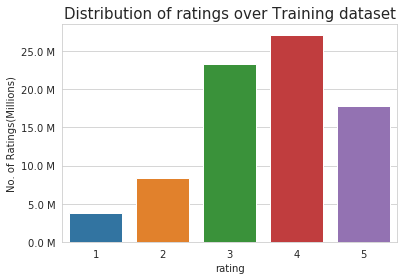Add new column (week day) to the data set for analysis.

In :
# It is used to skip the warning ''SettingWithCopyWarning''..
pd.options.mode.chained_assignment = None  # default='warn'

train_df['day_of_week'] = train_df.date.dt.weekday_name

train_df.tail()

Out:
movie user rating date day_of_week
80384400 12074 2033618 4 2005-08-08 Monday
80384401 862 1797061 3 2005-08-08 Monday
80384402 10986 1498715 5 2005-08-08 Monday
80384403 14861 500016 4 2005-08-08 Monday
80384404 5926 1044015 5 2005-08-08 Monday

### 3.3.2 Number of Ratings per a month

In :
ax = train_df.resample('m', on='date')['rating'].count().plot()
ax.set_title('No of ratings per month (Training data)')
plt.xlabel('Month')
plt.ylabel('No of ratings(per month)')
ax.set_yticklabels([human(item, 'M') for item in ax.get_yticks()])
plt.show()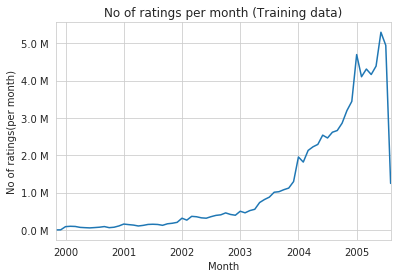### 3.3.3 Analysis on the Ratings given by user

In :
no_of_rated_movies_per_user = train_df.groupby(by='user')['rating'].count().sort_values(ascending=False)


Out:
user
305344     17112
2439493    15896
387418     15402
1639792     9767
1461435     9447
Name: rating, dtype: int64
In :
no_of_rated_movies_per_user.describe()

Out:
count    405041.000000
mean        198.459921
std         290.793238
min           1.000000
25%          34.000000
50%          89.000000
75%         245.000000
max       17112.000000
Name: rating, dtype: float64
In :
plt.plot(no_of_rated_movies_per_user.values)

Out:
[<matplotlib.lines.Line2D at 0x7fd3e660a518>]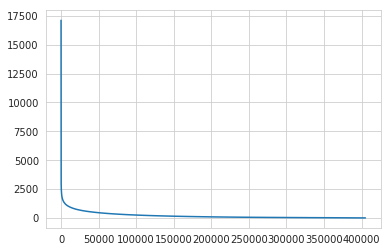In :
plt.plot(no_of_rated_movies_per_user.values[:100])

Out:
[<matplotlib.lines.Line2D at 0x7fd3e66abe48>]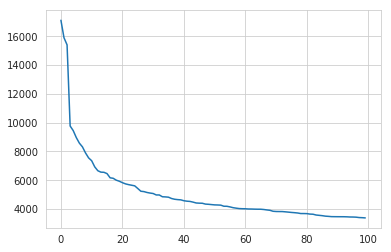In :
tyfig = plt.figure(figsize=plt.figaspect(.5))

ax1 = plt.subplot(121)
plt.xlabel('No of ratings by user')
plt.title("PDF")

ax2 = plt.subplot(122)
plt.xlabel('No of ratings by user')
plt.title('CDF')

plt.show()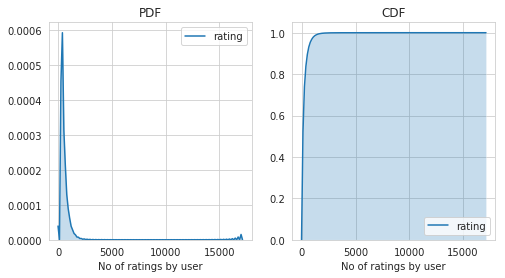In :
no_of_rated_movies_per_user.describe()

Out:
count    405041.000000
mean        198.459921
std         290.793238
min           1.000000
25%          34.000000
50%          89.000000
75%         245.000000
max       17112.000000
Name: rating, dtype: float64

There, is something interesting going on with the quantiles..

In :
quantiles = no_of_rated_movies_per_user.quantile(np.arange(0,1.01,0.01), interpolation='higher')

In :


In :
plt.title("Quantiles and their Values")
quantiles.plot()
# quantiles with 0.05 difference
plt.scatter(x=quantiles.index[::5], y=quantiles.values[::5], c='orange', label="quantiles with 0.05 intervals")
# quantiles with 0.25 difference
plt.scatter(x=quantiles.index[::25], y=quantiles.values[::25], c='m', label = "quantiles with 0.25 intervals")
plt.ylabel('No of ratings by user')
plt.xlabel('Value at the quantile')
plt.legend(loc='best')

# annotate the 25th, 50th, 75th and 100th percentile values....
for x,y in zip(quantiles.index[::25], quantiles[::25]):
plt.annotate(s="({} , {})".format(x,y), xy=(x,y), xytext=(x-0.05, y+500)
,fontweight='bold')

plt.show()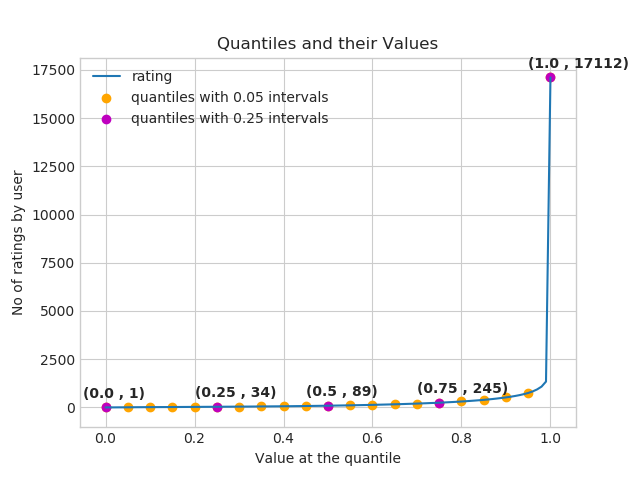In :
quantiles[::5]

Out:
0.00        1
0.05        7
0.10       15
0.15       21
0.20       27
0.25       34
0.30       41
0.35       50
0.40       60
0.45       73
0.50       89
0.55      109
0.60      133
0.65      163
0.70      199
0.75      245
0.80      307
0.85      392
0.90      520
0.95      749
1.00    17112
Name: rating, dtype: int64

how many ratings at the last 5% of all ratings??

In :
print('\n No of ratings at last 5 percentile : {}\n'.format(sum(no_of_rated_movies_per_user>= 749)) )

 No of ratings at last 5 percentile : 20305



### 3.3.4 Analysis of ratings of a movie given by a user

In :
no_of_ratings_per_movie = train_df.groupby(by='movie')['rating'].count().sort_values(ascending=False)

fig = plt.figure(figsize=plt.figaspect(.5))
ax = plt.gca()
plt.plot(no_of_ratings_per_movie.values)
plt.title('# RATINGS per Movie')
plt.xlabel('Movie')
plt.ylabel('No of Users who rated a movie')
ax.set_xticklabels([])

plt.show()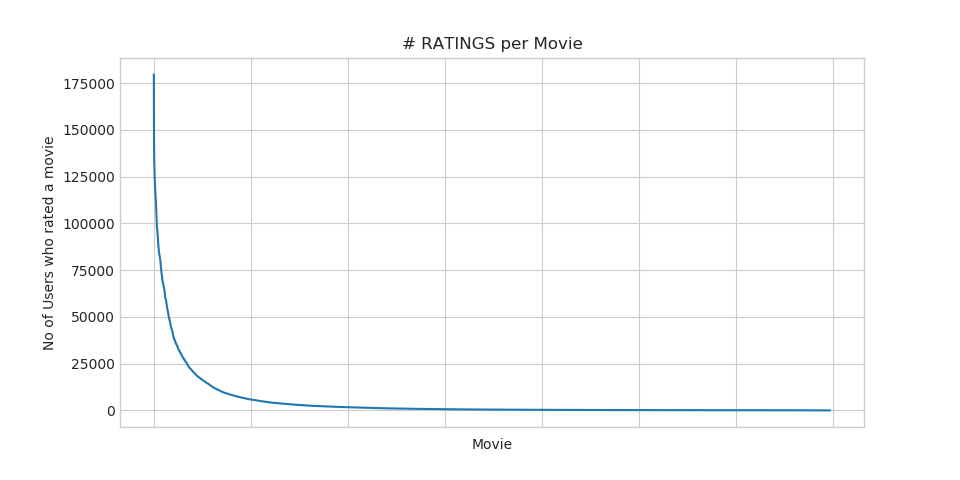• It is very skewed.. just like nunmber of ratings given per user.
- There are some movies (which are very popular) which are rated by huge number of users.

- But most of the movies(like 90%) got some hundereds of ratings.

### 3.3.5 Number of ratings on each day of the week

In :
fig, ax = plt.subplots()
sns.countplot(x='day_of_week', data=train_df, ax=ax)
plt.title('No of ratings on each day...')
plt.ylabel('Total no of ratings')
plt.xlabel('')
ax.set_yticklabels([human(item, 'M') for item in ax.get_yticks()])
plt.show()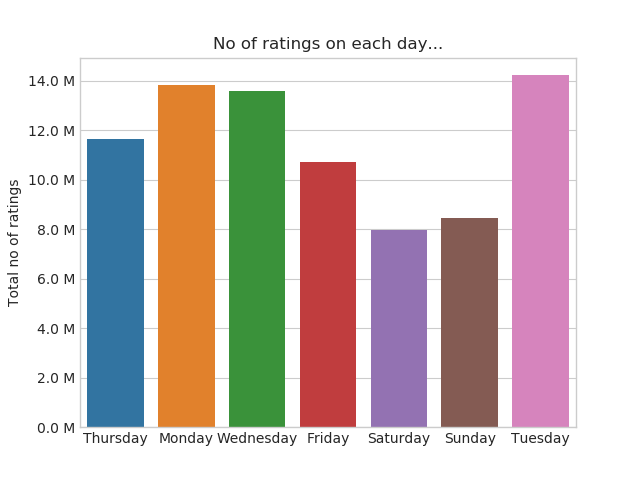In :
start = datetime.now()
fig = plt.figure(figsize=plt.figaspect(.45))
sns.boxplot(y='rating', x='day_of_week', data=train_df)
plt.show()
print(datetime.now() - start)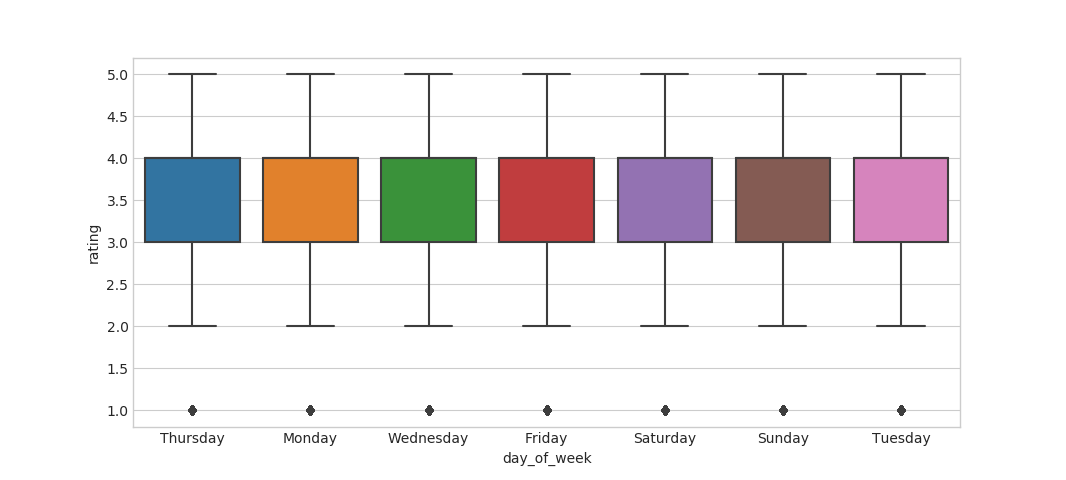0:01:10.003761

In :
avg_week_df = train_df.groupby(by=['day_of_week'])['rating'].mean()
print(" AVerage ratings")
print("-"*30)
print(avg_week_df)
print("\n")

 AVerage ratings
------------------------------
day_of_week
Friday       3.585274
Monday       3.577250
Saturday     3.591791
Sunday       3.594144
Thursday     3.582463
Tuesday      3.574438
Wednesday    3.583751
Name: rating, dtype: float64



### 3.3.6 Creating sparse matrix from data frame#### 3.3.6.1 Creating sparse matrix from train data frame

In :
start = datetime.now()
if os.path.isfile('/content/drive/My Drive/Netflix_recommender/data_folder/train_sparse_matrix.npz'):
print("It is present in your pwd, getting it from disk....")
# just get it from the disk instead of computing it
print("DONE..")
else:
print("We are creating sparse_matrix from the dataframe..")
# create sparse_matrix and store it for after usage.
# csr_matrix(data_values, (row_index, col_index), shape_of_matrix)
# It should be in such a way that, MATRIX[row, col] = data
train_sparse_matrix = sparse.csr_matrix((train_df.rating.values, (train_df.user.values,
train_df.movie.values)),)

print('Done. It\'s shape is : (user, movie) : ',train_sparse_matrix.shape)
print('Saving it into disk for furthur usage..')
# save it into disk
sparse.save_npz("train_sparse_matrix.npz", train_sparse_matrix)
print('Done..\n')

print(datetime.now() - start)

It is present in your pwd, getting it from disk....
DONE..
0:00:04.955848


The Sparsity of Train Sparse Matrix

In :
us,mv = train_sparse_matrix.shape
elem = train_sparse_matrix.count_nonzero()

print("Sparsity Of Train matrix : {} % ".format(  (1-(elem/(us*mv))) * 100) )

Sparsity Of Train matrix : 99.8292709259195 %


#### 3.3.6.2 Creating sparse matrix from test data frame

In :
start = datetime.now()
if os.path.isfile('/content/drive/My Drive/Netflix_recommender/data_folder/test_sparse_matrix.npz'):
print("It is present in your pwd, getting it from disk....")
# just get it from the disk instead of computing it
print("DONE..")
else:
print("We are creating sparse_matrix from the dataframe..")
# create sparse_matrix and store it for after usage.
# csr_matrix(data_values, (row_index, col_index), shape_of_matrix)
# It should be in such a way that, MATRIX[row, col] = data
test_sparse_matrix = sparse.csr_matrix((test_df.rating.values, (test_df.user.values,
test_df.movie.values)))

print('Done. It\'s shape is : (user, movie) : ',test_sparse_matrix.shape)
print('Saving it into disk for furthur usage..')
# save it into disk
sparse.save_npz("test_sparse_matrix.npz", test_sparse_matrix)
print('Done..\n')

print(datetime.now() - start)

It is present in your pwd, getting it from disk....
DONE..
0:00:02.357853


The Sparsity of Test data Matrix

In :
us,mv = test_sparse_matrix.shape
elem = test_sparse_matrix.count_nonzero()

print("Sparsity Of Test matrix : {} % ".format(  (1-(elem/(us*mv))) * 100) )

Sparsity Of Test matrix : 99.95731772988694 %


### 3.3.7 Finding Global average of all movie ratings, Average rating per user, and Average rating per movie

In :
# get the user averages in dictionary (key: user_id/movie_id, value: avg rating)

def get_average_ratings(sparse_matrix, of_users):

# average ratings of user/axes
ax = 1 if of_users else 0 # 1 - User axes,0 - Movie axes

# ".A1" is for converting Column_Matrix to 1-D numpy array
sum_of_ratings = sparse_matrix.sum(axis=ax).A1
# Boolean matrix of ratings ( whether a user rated that movie or not)
is_rated = sparse_matrix!=0
# no of ratings that each user OR movie..
no_of_ratings = is_rated.sum(axis=ax).A1

# max_user  and max_movie ids in sparse matrix
u,m = sparse_matrix.shape
# creae a dictonary of users and their average ratigns..
average_ratings = { i : sum_of_ratings[i]/no_of_ratings[i]
for i in range(u if of_users else m)
if no_of_ratings[i] !=0}

# return that dictionary of average ratings
return average_ratings


#### 3.3.7.1 finding global average of all movie ratings

In :
train_averages = dict()
# get the global average of ratings in our train set.
train_global_average = train_sparse_matrix.sum()/train_sparse_matrix.count_nonzero()
train_averages['global'] = train_global_average
train_averages

Out:
{'global': 3.582890686321557}

#### 3.3.7.2 finding average rating per user

In :
train_averages['user'] = get_average_ratings(train_sparse_matrix, of_users=True)
print('\nAverage rating of user 10 :',train_averages['user'])

Average rating of user 10 : 3.3781094527363185


#### 3.3.7.3 finding average rating per movie

In :
train_averages['movie'] =  get_average_ratings(train_sparse_matrix, of_users=False)
print('\n AVerage rating of movie 15 :',train_averages['movie'])

 AVerage rating of movie 15 : 3.3038461538461537


#### 3.3.7.4 PDF's & CDF's of Avg.Ratings of Users & Movies (In Train Data)

In :
start = datetime.now()
# draw pdfs for average rating per user and average
fig, (ax1, ax2) = plt.subplots(nrows=1, ncols=2, figsize=plt.figaspect(.5))
fig.suptitle('Avg Ratings per User and per Movie', fontsize=15)

ax1.set_title('Users-Avg-Ratings')
# get the list of average user ratings from the averages dictionary..
user_averages = [rat for rat in train_averages['user'].values()]
sns.distplot(user_averages, ax=ax1, hist=False,
kde_kws=dict(cumulative=True), label='Cdf')
sns.distplot(user_averages, ax=ax1, hist=False,label='Pdf')

ax2.set_title('Movies-Avg-Rating')
# get the list of movie_average_ratings from the dictionary..
movie_averages = [rat for rat in train_averages['movie'].values()]
sns.distplot(movie_averages, ax=ax2, hist=False,
kde_kws=dict(cumulative=True), label='Cdf')
sns.distplot(movie_averages, ax=ax2, hist=False, label='Pdf')

plt.show()
print(datetime.now() - start)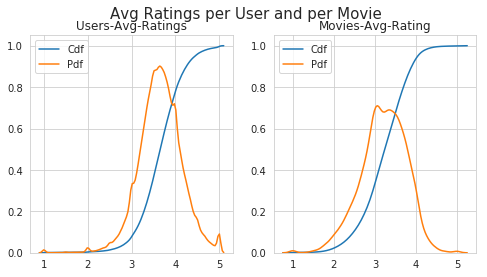0:00:41.239658


### 3.3.8 Cold Start problem

#### 3.3.8.1 Cold Start problem with Users

In :
total_users = len(np.unique(df.user))
users_train = len(train_averages['user'])
new_users = total_users - users_train

print('\nTotal number of Users  :', total_users)
print('\nNumber of Users in Train data :', users_train)
print("\nNo of Users that didn't appear in train data: {}({} %) \n ".format(new_users,
np.round((new_users/total_users)*100, 2)))

Total number of Users  : 480189

Number of Users in Train data : 405041

No of Users that didn't appear in train data: 75148(15.65 %)



We might have to handle new users ( 75148 ) who didn't appear in train data.

#### 3.3.8.2 Cold Start problem with Movies

In :
total_movies = len(np.unique(df.movie))
movies_train = len(train_averages['movie'])
new_movies = total_movies - movies_train

print('\nTotal number of Movies  :', total_movies)
print('\nNumber of Users in Train data :', movies_train)
print("\nNo of Movies that didn't appear in train data: {}({} %) \n ".format(new_movies,
np.round((new_movies/total_movies)*100, 2)))

Total number of Movies  : 17770

Number of Users in Train data : 17424

No of Movies that didn't appear in train data: 346(1.95 %)



We might have to handle 346 movies (small comparatively) in test data

## 3.4 Computing Similarity matrices

### 3.4.1 Computing User-User Similarity matrix

1. Calculating User User Similarity_Matrix is not very easy(unless you have huge Computing Power and lots of time) because of number of. usersbeing lare.

• You can try if you want to. Your system could crash or the program stops with Memory Error

#### 3.4.1.1 Trying with all dimensions (17k dimensions per user)

In :
from sklearn.metrics.pairwise import cosine_similarity

def compute_user_similarity(sparse_matrix, compute_for_few=False, top = 100, verbose=False, verb_for_n_rows = 20,
draw_time_taken=True):
no_of_users, _ = sparse_matrix.shape
# get the indices of  non zero rows(users) from our sparse matrix
row_ind, col_ind = sparse_matrix.nonzero()
row_ind = sorted(set(row_ind)) # we don't have to
time_taken = list() #  time taken for finding similar users for an user..

# we create rows, cols, and data lists.., which can be used to create sparse matrices
rows, cols, data = list(), list(), list()
if verbose: print("Computing top",top,"similarities for each user..")

start = datetime.now()
temp = 0

for row in row_ind[:top] if compute_for_few else row_ind:
temp = temp+1
prev = datetime.now()

# get the similarity row for this user with all other users
sim = cosine_similarity(sparse_matrix.getrow(row), sparse_matrix).ravel()
# We will get only the top ''top'' most similar users and ignore rest of them..
top_sim_ind = sim.argsort()[-top:]
top_sim_val = sim[top_sim_ind]

# add them to our rows, cols and data
rows.extend([row]*top)
cols.extend(top_sim_ind)
data.extend(top_sim_val)
time_taken.append(datetime.now().timestamp() - prev.timestamp())
if verbose:
if temp%verb_for_n_rows == 0:
print("computing done for {} users [  time elapsed : {}  ]"
.format(temp, datetime.now()-start))

# lets create sparse matrix out of these and return it
if verbose: print('Creating Sparse matrix from the computed similarities')
#return rows, cols, data

if draw_time_taken:
plt.plot(time_taken, label = 'time taken for each user')
plt.plot(np.cumsum(time_taken), label='Total time')
plt.legend(loc='best')
plt.xlabel('User')
plt.ylabel('Time (seconds)')
plt.show()

return sparse.csr_matrix((data, (rows, cols)), shape=(no_of_users, no_of_users)), time_taken

In :
start = datetime.now()
u_u_sim_sparse, _ = compute_user_similarity(train_sparse_matrix, compute_for_few=True, top = 100,
verbose=True)
print("-"*100)
print("Time taken :",datetime.now()-start)


#### 3.4.1.2 Trying with reduced dimensions (Using TruncatedSVD for dimensionality reduction of user vector)

• We have 405,041 users in out training set and computing similarities between them..( 17K dimensional vector..) is time consuming..
• From above plot, It took roughly 8.88 sec for computing simlilar users for one user
• We have 405,041 users with us in training set.
• ${ 405041 \times 8.88 = 3596764.08 \sec } = 59946.068 \min = 999.101133333 \text{ hours} = 41.629213889 \text{ days}...$

• Even if we run on 4 cores parallelly (a typical system now a days), It will still take almost 10 and 1/2 days.

IDEA: Instead, we will try to reduce the dimentsions using SVD, so that it might speed up the process...

In :
from datetime import datetime
from sklearn.decomposition import TruncatedSVD

start = datetime.now()

# initilaize the algorithm with some parameters..
# All of them are default except n_components. n_itr is for Randomized SVD solver.
netflix_svd = TruncatedSVD(n_components=500, algorithm='randomized', random_state=15)
trunc_svd = netflix_svd.fit_transform(train_sparse_matrix)

print(datetime.now()-start)

0:29:07.069783


Here,

• $\sum \longleftarrow$ (netflix_svd.singular_values_ )
• $\bigvee^T \longleftarrow$ (netflix_svd.components_)
• $\bigcup$ is not returned. instead Projection_of_X onto the new vectorspace is returned.
• It uses randomized svd internally, which returns All 3 of them saperately. Use that instead..
In :
expl_var = np.cumsum(netflix_svd.explained_variance_ratio_)

In :
fig, (ax1, ax2) = plt.subplots(nrows=1, ncols=2, figsize=plt.figaspect(.5))

ax1.set_ylabel("Variance Explained", fontsize=15)
ax1.set_xlabel("# Latent Facors", fontsize=15)
ax1.plot(expl_var)
# annote some (latentfactors, expl_var) to make it clear
ind = [1, 2,4,8,20, 60, 100, 200, 300, 400, 500]
ax1.scatter(x = [i-1 for i in ind], y = expl_var[[i-1 for i in ind]], c='#ff3300')
for i in ind:
ax1.annotate(s ="({}, {})".format(i,  np.round(expl_var[i-1], 2)), xy=(i-1, expl_var[i-1]),
xytext = ( i+20, expl_var[i-1] - 0.01), fontweight='bold')

change_in_expl_var = [expl_var[i+1] - expl_var[i] for i in range(len(expl_var)-1)]
ax2.plot(change_in_expl_var)

ax2.set_ylabel("Gain in Var_Expl with One Additional LF", fontsize=10)
ax2.yaxis.set_label_position("right")
ax2.set_xlabel("# Latent Facors", fontsize=20)

plt.show()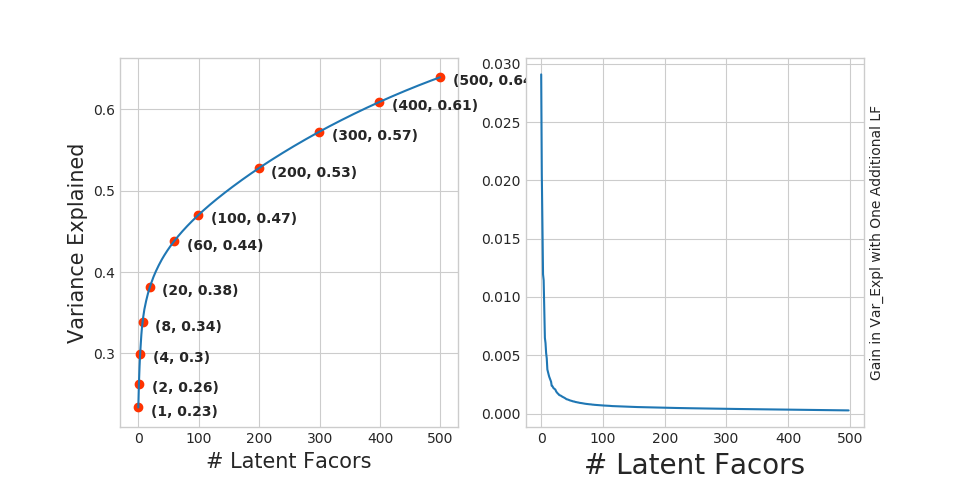In :
for i in ind:
print("({}, {})".format(i, np.round(expl_var[i-1], 2)))

(1, 0.23)
(2, 0.26)
(4, 0.3)
(8, 0.34)
(20, 0.38)
(60, 0.44)
(100, 0.47)
(200, 0.53)
(300, 0.57)
(400, 0.61)
(500, 0.64)


I think 500 dimensions is good enough

• By just taking (20 to 30) latent factors, explained variance that we could get is 20 %.

• To take it to 60%, we have to take almost 400 latent factors. It is not fare.

• It basically is the gain of variance explained, if we add one additional latent factor to it.
• By adding one by one latent factore too it, the _gain in expained variance with that addition is decreasing. (Obviously, because they are sorted that way).
• LHS Graph:
• x --- ( No of latent factos ),
• y --- ( The variance explained by taking x latent factors)
• More decrease in the line (RHS graph) :
• We are getting more expained variance than before.
• Less decrease in that line (RHS graph) :
• We are not getting benifitted from adding latent factor furthur. This is what is shown in the plots.
• RHS Graph:
• x --- ( No of latent factors ),
• y --- ( Gain n Expl_Var by taking one additional latent factor)
In :
# Let's project our Original U_M matrix into into 500 Dimensional space...
start = datetime.now()
trunc_matrix = train_sparse_matrix.dot(netflix_svd.components_.T)
print(datetime.now()- start)

0:00:45.670265

In :
type(trunc_matrix), trunc_matrix.shape

Out:
(numpy.ndarray, (2649430, 500))
• Let's convert this to actual sparse matrix and store it for future purposes
In :
if not os.path.isfile('/content/drive/My Drive/Netflix_recommender/data_folder/trunc_sparse_matrix.npz'):
# create that sparse sparse matrix
trunc_sparse_matrix = sparse.csr_matrix(trunc_matrix)
# Save this truncated sparse matrix for later usage..
sparse.save_npz('trunc_sparse_matrix', trunc_sparse_matrix)
else:

In :
trunc_sparse_matrix.shape

Out:
(2649430, 500)
In :
start = datetime.now()
trunc_u_u_sim_matrix, _ = compute_user_similarity(trunc_sparse_matrix, compute_for_few=True, top=50, verbose=True,
verb_for_n_rows=10)
print("-"*50)
print("time:",datetime.now()-start)

Computing top 50 similarities for each user..
computing done for 10 users [  time elapsed : 0:02:09.746324  ]
computing done for 20 users [  time elapsed : 0:04:16.017768  ]
computing done for 30 users [  time elapsed : 0:06:20.861163  ]
computing done for 40 users [  time elapsed : 0:08:24.933316  ]
computing done for 50 users [  time elapsed : 0:10:28.861485  ]
Creating Sparse matrix from the computed similarities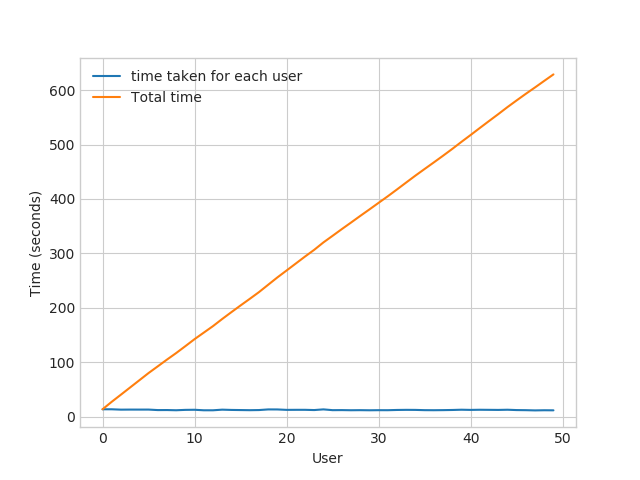--------------------------------------------------
time: 0:10:52.658092


: This is taking more time for each user than Original one.

• from above plot, It took almost 12.18 for computing simlilar users for one user
• We have 405041 users with us in training set.
• ${ 405041 \times 12.18 ==== 4933399.38 \sec } ==== 82223.323 \min ==== 1370.388716667 \text{ hours} ==== 57.099529861 \text{ days}...$

• Even we run on 4 cores parallelly (a typical system now a days), It will still take almost (14 - 15) days.
• Why did this happen...??
- Just think about it. It's not that difficult.



---------------------------------( sparse & dense..................get it ?? )-----------------------------------

Is there any other way to compute user user similarity..??

-An alternative is to compute similar users for a particular user, whenenver required (ie., Run time)

- We maintain a binary Vector for users, which tells us whether we already computed or not..
- ***If not*** :
- Compute top (let's just say, 1000) most similar users for this given user, and add this to our datastructure, so that we can just access it(similar users) without recomputing it again.
-
- ***If It is already Computed***:
- Just get it directly from our datastructure, which has that information.
- In production time, We might have to recompute similarities, if it is computed a long time ago. Because user preferences changes over time. If we could maintain some kind of Timer, which when expires, we have to update it ( recompute it ).
-
- ***Which datastructure to use:***
- It is purely implementation dependant.
- One simple method is to maintain a **Dictionary Of Dictionaries**.
-
- **key    :** _userid_
- __value__: _Again a dictionary_
- __key__  : _Similar User_
- __value__: _Similarity Value_

### 3.4.2 Computing Movie-Movie Similarity matrix

In :
start = datetime.now()
if not os.path.isfile('/content/drive/My Drive/Netflix_recommender/data_folder/m_m_sim_sparse.npz'):
print("It seems you don't have that file. Computing movie_movie similarity...")
start = datetime.now()
m_m_sim_sparse = cosine_similarity(X=train_sparse_matrix.T, dense_output=False)
print("Done..")
# store this sparse matrix in disk before using it. For future purposes.
print("Saving it to disk without the need of re-computing it again.. ")
sparse.save_npz("m_m_sim_sparse.npz", m_m_sim_sparse)
print("Done..")
else:
print("It is there, We will get it.")
print("Done ...")

print("It's a ",m_m_sim_sparse.shape," dimensional matrix")

print(datetime.now() - start)

In :
m_m_sim_sparse.shape

Out:
(17771, 17771)
• Even though we have similarity measure of each movie, with all other movies, We generally don't care much about least similar movies.
• Most of the times, only top_xxx similar items matters. It may be 10 or 100.
• We take only those top similar movie ratings and store them in a saperate dictionary.
In :
movie_ids = np.unique(m_m_sim_sparse.nonzero())

In :
start = datetime.now()
similar_movies = dict()
for movie in movie_ids:
# get the top similar movies and store them in the dictionary
sim_movies = m_m_sim_sparse[movie].toarray().ravel().argsort()[::-1][1:]
similar_movies[movie] = sim_movies[:100]
print(datetime.now() - start)

# just testing similar movies for movie_15
similar_movies

0:00:33.411700

Out:
array([ 8279,  8013, 16528,  5927, 13105, 12049,  4424, 10193, 17590,
4549,  3755,   590, 14059, 15144, 15054,  9584,  9071,  6349,
16402,  3973,  1720,  5370, 16309,  9376,  6116,  4706,  2818,
778, 15331,  1416, 12979, 17139, 17710,  5452,  2534,   164,
15188,  8323,  2450, 16331,  9566, 15301, 13213, 14308, 15984,
10597,  6426,  5500,  7068,  7328,  5720,  9802,   376, 13013,
8003, 10199,  3338, 15390,  9688, 16455, 11730,  4513,   598,
12762,  2187,   509,  5865,  9166, 17115, 16334,  1942,  7282,
17584,  4376,  8988,  8873,  5921,  2716, 14679, 11947, 11981,
4649,   565, 12954, 10788, 10220, 10963,  9427,  1690,  5107,
7859,  5969,  1510,  2429,   847,  7845,  6410, 13931,  9840,
3706])

### 3.4.3 Finding most similar movies using similarity matrix

Does Similarity really works as the way we expected...?
Let's pick some random movie and check for its similar movies....

In :
# First Let's load the movie details into soe dataframe..
# movie details are in 'netflix/movie_titles.csv'

names=['movie_id', 'year_of_release', 'title'], verbose=True,
index_col = 'movie_id', encoding = "ISO-8859-1")


Tokenization took: 4.50 ms
Type conversion took: 165.72 ms
Parser memory cleanup took: 0.01 ms

Out:
year_of_release title
movie_id
1 2003.0 Dinosaur Planet
2 2004.0 Isle of Man TT 2004 Review
3 1997.0 Character
4 1994.0 Paula Abdul's Get Up & Dance
5 2004.0 The Rise and Fall of ECW

Similar Movies for 'Vampire Journals'

In :
mv_id = 67

print("\nMovie ----->",movie_titles.loc[mv_id].values)

print("\nIt has {} Ratings from users.".format(train_sparse_matrix[:,mv_id].getnnz()))

print("\nWe have {} movies which are similarto this  and we will get only top most..".format(m_m_sim_sparse[:,mv_id].getnnz()))

Movie -----> Vampire Journals

It has 270 Ratings from users.

We have 17284 movies which are similarto this  and we will get only top most..

In :
similarities = m_m_sim_sparse[mv_id].toarray().ravel()

similar_indices = similarities.argsort()[::-1][1:]

similarities[similar_indices]

sim_indices = similarities.argsort()[::-1][1:] # It will sort and reverse the array and ignore its similarity (ie.,1)
# and return its indices(movie_ids)

In :
plt.plot(similarities[sim_indices], label='All the ratings')
plt.plot(similarities[sim_indices[:100]], label='top 100 similar movies')
plt.title("Similar Movies of {}(movie_id)".format(mv_id), fontsize=20)
plt.xlabel("Movies (Not Movie_Ids)", fontsize=15)
plt.ylabel("Cosine Similarity",fontsize=15)
plt.legend()
plt.show()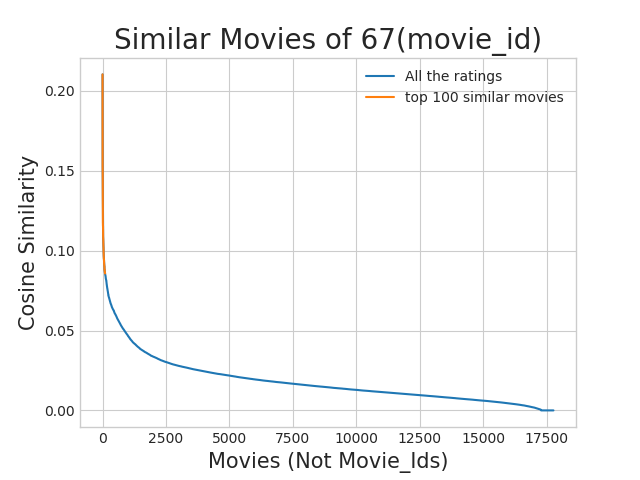Top 10 similar movies

In :
movie_titles.loc[sim_indices[:10]]

Out:
year_of_release title
movie_id
323 1999.0 Modern Vampires
4044 1998.0 Subspecies 4: Bloodstorm
1688 1993.0 To Sleep With a Vampire
13962 2001.0 Dracula: The Dark Prince
12053 1993.0 Dracula Rising
16279 2002.0 Vampires: Los Muertos
4667 1996.0 Vampirella
1900 1997.0 Club Vampire
13873 2001.0 The Breed
15867 2003.0 Dracula II: Ascension

Similarly, we can find similar users and compare how similar they are.

# 4. Machine Learning ModelsIn :
def get_sample_sparse_matrix(sparse_matrix, no_users, no_movies, path, verbose = True):
"""
It will get it from the ''path'' if it is present  or It will create
and store the sampled sparse matrix in the path specified.
"""

# get (row, col) and (rating) tuple from sparse_matrix...
row_ind, col_ind, ratings = sparse.find(sparse_matrix)
users = np.unique(row_ind)
movies = np.unique(col_ind)

print("Original Matrix : (users, movies) -- ({} {})".format(len(users), len(movies)))
print("Original Matrix : Ratings -- {}\n".format(len(ratings)))

# It just to make sure to get same sample everytime we run this program..
# and pick without replacement....
np.random.seed(15)
sample_users = np.random.choice(users, no_users, replace=False)
sample_movies = np.random.choice(movies, no_movies, replace=False)
# get the boolean mask or these sampled_items in originl row/col_inds..
np.isin(col_ind, sample_movies) )

shape=(max(sample_users)+1, max(sample_movies)+1))

if verbose:
print("Sampled Matrix : (users, movies) -- ({} {})".format(len(sample_users), len(sample_movies)))
print("Sampled Matrix : Ratings --", format(ratings[mask].shape))

print('Saving it into disk for furthur usage..')
# save it into disk
sparse.save_npz(path, sample_sparse_matrix)
if verbose:
print('Done..\n')

return sample_sparse_matrix


## 4.1 Sampling Data

### 4.1.1 Build sample train data from the train data

In :
start = datetime.now()
path = "/content/drive/My Drive/Netflix_recommender/sample_train_sparse_matrix.npz"
if os.path.isfile(path):
print("It is present in your pwd, getting it from disk....")
# just get it from the disk instead of computing it
print("DONE..")
else:
# get 10k users and 1k movies from available data
sample_train_sparse_matrix = get_sample_sparse_matrix(train_sparse_matrix, no_users=13000, no_movies=1500,
path = path)

print(datetime.now() - start)

Original Matrix : (users, movies) -- (405041 17424)
Original Matrix : Ratings -- 80384405

Sampled Matrix : (users, movies) -- (13000 1500)
Sampled Matrix : Ratings -- 239403
Saving it into disk for furthur usage..
Done..

0:01:04.277437


### 4.1.2 Build sample test data from the test data

In :
start = datetime.now()

path = "/content/drive/My Drive/Netflix_recommender/sample_test_sparse_matrix.npz"
if os.path.isfile(path):
print("It is present in your pwd, getting it from disk....")
# just get it from the disk instead of computing it
print("DONE..")
else:
# get 5k users and 500 movies from available data
sample_test_sparse_matrix = get_sample_sparse_matrix(test_sparse_matrix, no_users=5000, no_movies=500,
path = "/content/drive/My Drive/Netflix_recommender/sample_test_sparse_matrix.npz")
print(datetime.now() - start)

Original Matrix : (users, movies) -- (349312 17757)
Original Matrix : Ratings -- 20096102

Sampled Matrix : (users, movies) -- (5000 500)
Sampled Matrix : Ratings -- 7333
Saving it into disk for furthur usage..
Done..

0:00:14.324827


## 4.2 Finding Global Average of all movie ratings, Average rating per User, and Average rating per Movie (from sampled train)

In :
sample_train_averages = dict()


### 4.2.1 Finding Global Average of all movie ratings

In :
# get the global average of ratings in our train set.
global_average = sample_train_sparse_matrix.sum()/sample_train_sparse_matrix.count_nonzero()
sample_train_averages['global'] = global_average
sample_train_averages

Out:
{'global': 3.5437651157253667}

### 4.2.2 Finding Average rating per User

In :
sample_train_averages['user'] = get_average_ratings(sample_train_sparse_matrix, of_users=True)
print('\nAverage rating of user 1515220 :',sample_train_averages['user'])

Average rating of user 1515220 : 3.953488372093023


### 4.2.3 Finding Average rating per Movie

In :
sample_train_averages['movie'] =  get_average_ratings(sample_train_sparse_matrix, of_users=False)
print('\n AVerage rating of movie 15153 :',sample_train_averages['movie'])

 AVerage rating of movie 15153 : 2.6


## 4.3 Featurizing data

In :
print('\n No of ratings in Our Sampled train matrix is : {}\n'.format(sample_train_sparse_matrix.count_nonzero()))
print('\n No of ratings in Our Sampled test  matrix is : {}\n'.format(sample_test_sparse_matrix.count_nonzero()))

 No of ratings in Our Sampled train matrix is : 239403

No of ratings in Our Sampled test  matrix is : 7333



### 4.3.1 Featurizing data for regression problem

#### 4.3.1.1 Featurizing train data

In :
# get users, movies and ratings from our samples train sparse matrix
sample_train_users, sample_train_movies, sample_train_ratings = sparse.find(sample_train_sparse_matrix)

In :
############################################################
# It took me almost 10 hours to prepare this train dataset.#
############################################################
start = datetime.now()
if os.path.isfile('sample/small/reg_train.csv'):
print("File already exists you don't have to prepare again..." )
else:
print('preparing {} tuples for the dataset..\n'.format(len(sample_train_ratings)))
with open('sample/small/reg_train.csv', mode='w') as reg_data_file:
count = 0
for (user, movie, rating)  in zip(sample_train_users, sample_train_movies, sample_train_ratings):
st = datetime.now()
#     print(user, movie)
#--------------------- Ratings of "movie" by similar users of "user" ---------------------
# compute the similar Users of the "user"
user_sim = cosine_similarity(sample_train_sparse_matrix[user], sample_train_sparse_matrix).ravel()
top_sim_users = user_sim.argsort()[::-1][1:] # we are ignoring 'The User' from its similar users.
# get the ratings of most similar users for this movie
top_ratings = sample_train_sparse_matrix[top_sim_users, movie].toarray().ravel()
# we will make it's length "5" by adding movie averages to .
top_sim_users_ratings = list(top_ratings[top_ratings != 0][:5])
top_sim_users_ratings.extend([sample_train_averages['movie'][movie]]*(5 - len(top_sim_users_ratings)))
#     print(top_sim_users_ratings, end=" ")

#--------------------- Ratings by "user"  to similar movies of "movie" ---------------------
# compute the similar movies of the "movie"
movie_sim = cosine_similarity(sample_train_sparse_matrix[:,movie].T, sample_train_sparse_matrix.T).ravel()
top_sim_movies = movie_sim.argsort()[::-1][1:] # we are ignoring 'The User' from its similar users.
# get the ratings of most similar movie rated by this user..
top_ratings = sample_train_sparse_matrix[user, top_sim_movies].toarray().ravel()
# we will make it's length "5" by adding user averages to.
top_sim_movies_ratings = list(top_ratings[top_ratings != 0][:5])
top_sim_movies_ratings.extend([sample_train_averages['user'][user]]*(5-len(top_sim_movies_ratings)))
#     print(top_sim_movies_ratings, end=" : -- ")

#-----------------prepare the row to be stores in a file-----------------#
row = list()
row.append(user)
row.append(movie)
# Now add the other features to this data...
row.append(sample_train_averages['global']) # first feature
# next 5 features are similar_users "movie" ratings
row.extend(top_sim_users_ratings)
# next 5 features are "user" ratings for similar_movies
row.extend(top_sim_movies_ratings)
# Avg_user rating
row.append(sample_train_averages['user'][user])
# Avg_movie rating
row.append(sample_train_averages['movie'][movie])

# finalley, The actual Rating of this user-movie pair...
row.append(rating)
count = count + 1

# add rows to the file opened..
reg_data_file.write(','.join(map(str, row)))
reg_data_file.write('\n')
if (count)%10000 == 0:
# print(','.join(map(str, row)))
print("Done for {} rows----- {}".format(count, datetime.now() - start))

print(datetime.now() - start)

preparing 129286 tuples for the dataset..

Done for 10000 rows----- 0:53:13.974716
Done for 20000 rows----- 1:47:58.228942
Done for 30000 rows----- 2:42:46.963119
Done for 40000 rows----- 3:36:44.807894
Done for 50000 rows----- 4:28:55.311500
Done for 60000 rows----- 5:24:18.493104
Done for 70000 rows----- 6:17:39.669922
Done for 80000 rows----- 7:11:23.970879
Done for 90000 rows----- 8:05:33.787770
Done for 100000 rows----- 9:00:25.463562
Done for 110000 rows----- 9:51:28.530010
Done for 120000 rows----- 10:42:05.382141
11:30:13.699183


Reading from the file to make a Train_dataframe

In :
reg_train = pd.read_csv('/content/drive/My Drive/Netflix_recommender/reg_train_25.csv', names = ['user', 'movie', 'GAvg', 'sur1', 'sur2', 'sur3', 'sur4', 'sur5','smr1', 'smr2', 'smr3', 'smr4', 'smr5', 'UAvg', 'MAvg', 'rating'], header=None)

Out:
user movie GAvg sur1 sur2 sur3 sur4 sur5 smr1 smr2 smr3 smr4 smr5 UAvg MAvg rating
0 174683 10 3.587581 5.0 5.0 3.0 4.0 4.0 3.0 5.0 4.0 3.0 2.0 3.882353 3.611111 5
1 233949 10 3.587581 4.0 4.0 5.0 1.0 3.0 2.0 3.0 2.0 3.0 3.0 2.692308 3.611111 3
2 555770 10 3.587581 4.0 5.0 4.0 4.0 5.0 4.0 2.0 5.0 4.0 4.0 3.795455 3.611111 4
3 767518 10 3.587581 2.0 5.0 4.0 4.0 3.0 5.0 5.0 4.0 4.0 3.0 3.884615 3.611111 5
4 894393 10 3.587581 3.0 5.0 4.0 4.0 3.0 4.0 4.0 4.0 4.0 4.0 4.000000 3.611111 4

• GAvg : Average rating of all the ratings
• Similar users rating of this movie:
• sur1, sur2, sur3, sur4, sur5 ( top 5 similar users who rated that movie.. )
• Similar movies rated by this user:
• smr1, smr2, smr3, smr4, smr5 ( top 5 similar movies rated by this movie.. )
• UAvg : User's Average rating
• MAvg : Average rating of this movie
• rating : Rating of this movie by this user.

#### 4.3.1.2 Featurizing test data

In :
# get users, movies and ratings from the Sampled Test
sample_test_users, sample_test_movies, sample_test_ratings = sparse.find(sample_test_sparse_matrix)

In :
sample_train_averages['global']

Out:
3.581679377504138
In :
start = datetime.now()

if os.path.isfile('sample/small/reg_test.csv'):
else:

print('preparing {} tuples for the dataset..\n'.format(len(sample_test_ratings)))
with open('sample/small/reg_test.csv', mode='w') as reg_data_file:
count = 0
for (user, movie, rating)  in zip(sample_test_users, sample_test_movies, sample_test_ratings):
st = datetime.now()

#--------------------- Ratings of "movie" by similar users of "user" ---------------------
#print(user, movie)
try:
# compute the similar Users of the "user"
user_sim = cosine_similarity(sample_train_sparse_matrix[user], sample_train_sparse_matrix).ravel()
top_sim_users = user_sim.argsort()[::-1][1:] # we are ignoring 'The User' from its similar users.
# get the ratings of most similar users for this movie
top_ratings = sample_train_sparse_matrix[top_sim_users, movie].toarray().ravel()
# we will make it's length "5" by adding movie averages to .
top_sim_users_ratings = list(top_ratings[top_ratings != 0][:5])
top_sim_users_ratings.extend([sample_train_averages['movie'][movie]]*(5 - len(top_sim_users_ratings)))
# print(top_sim_users_ratings, end="--")

except (IndexError, KeyError):
# It is a new User or new Movie or there are no ratings for given user for top similar movies...
########## Cold STart Problem ##########
top_sim_users_ratings.extend([sample_train_averages['global']]*(5 - len(top_sim_users_ratings)))
#print(top_sim_users_ratings)
except:
print(user, movie)
# we just want KeyErrors to be resolved. Not every Exception...
raise

#--------------------- Ratings by "user"  to similar movies of "movie" ---------------------
try:
# compute the similar movies of the "movie"
movie_sim = cosine_similarity(sample_train_sparse_matrix[:,movie].T, sample_train_sparse_matrix.T).ravel()
top_sim_movies = movie_sim.argsort()[::-1][1:] # we are ignoring 'The User' from its similar users.
# get the ratings of most similar movie rated by this user..
top_ratings = sample_train_sparse_matrix[user, top_sim_movies].toarray().ravel()
# we will make it's length "5" by adding user averages to.
top_sim_movies_ratings = list(top_ratings[top_ratings != 0][:5])
top_sim_movies_ratings.extend([sample_train_averages['user'][user]]*(5-len(top_sim_movies_ratings)))
#print(top_sim_movies_ratings)
except (IndexError, KeyError):
#print(top_sim_movies_ratings, end=" : -- ")
top_sim_movies_ratings.extend([sample_train_averages['global']]*(5-len(top_sim_movies_ratings)))
#print(top_sim_movies_ratings)
except :
raise

#-----------------prepare the row to be stores in a file-----------------#
row = list()
# add usser and movie name first
row.append(user)
row.append(movie)
row.append(sample_train_averages['global']) # first feature
#print(row)
# next 5 features are similar_users "movie" ratings
row.extend(top_sim_users_ratings)
#print(row)
# next 5 features are "user" ratings for similar_movies
row.extend(top_sim_movies_ratings)
#print(row)
# Avg_user rating
try:
row.append(sample_train_averages['user'][user])
except KeyError:
row.append(sample_train_averages['global'])
except:
raise
#print(row)
# Avg_movie rating
try:
row.append(sample_train_averages['movie'][movie])
except KeyError:
row.append(sample_train_averages['global'])
except:
raise
#print(row)
# finalley, The actual Rating of this user-movie pair...
row.append(rating)
#print(row)
count = count + 1

# add rows to the file opened..
reg_data_file.write(','.join(map(str, row)))
#print(','.join(map(str, row)))
reg_data_file.write('\n')
if (count)%1000 == 0:
#print(','.join(map(str, row)))
print("Done for {} rows----- {}".format(count, datetime.now() - start))
print("",datetime.now() - start)

preparing 7333 tuples for the dataset..

Done for 1000 rows----- 0:04:29.293783
Done for 2000 rows----- 0:08:57.208002
Done for 3000 rows----- 0:13:30.333223
Done for 4000 rows----- 0:18:04.050813
Done for 5000 rows----- 0:22:38.671673
Done for 6000 rows----- 0:27:09.697009
Done for 7000 rows----- 0:31:41.933568
0:33:12.529731


Reading from the file to make a test dataframe

In :
reg_test_df = pd.read_csv('/content/drive/My Drive/Netflix_recommender/reg_test_25.csv', names = ['user', 'movie', 'GAvg', 'sur1', 'sur2', 'sur3', 'sur4', 'sur5',
'smr1', 'smr2', 'smr3', 'smr4', 'smr5',

Out:
user movie GAvg sur1 sur2 sur3 sur4 sur5 smr1 smr2 smr3 smr4 smr5 UAvg MAvg rating
0 808635 71 3.581679 3.581679 3.581679 3.581679 3.581679 3.581679 3.581679 3.581679 3.581679 3.581679 3.581679 3.581679 3.581679 5
1 941866 71 3.581679 3.581679 3.581679 3.581679 3.581679 3.581679 3.581679 3.581679 3.581679 3.581679 3.581679 3.581679 3.581679 4
2 1737912 71 3.581679 3.581679 3.581679 3.581679 3.581679 3.581679 3.581679 3.581679 3.581679 3.581679 3.581679 3.581679 3.581679 3
3 1849204 71 3.581679 3.581679 3.581679 3.581679 3.581679 3.581679 3.581679 3.581679 3.581679 3.581679 3.581679 3.581679 3.581679 4

• GAvg : Average rating of all the ratings
• Similar users rating of this movie:
• sur1, sur2, sur3, sur4, sur5 ( top 5 simiular users who rated that movie.. )
• Similar movies rated by this user:
• smr1, smr2, smr3, smr4, smr5 ( top 5 simiular movies rated by this movie.. )
• UAvg : User AVerage rating
• MAvg : Average rating of this movie
• rating : Rating of this movie by this user.

### 4.3.2 Transforming data for Surprise models

In :
from surprise import Reader, Dataset


#### 4.3.2.1 Transforming train data

• We can't give raw data (movie, user, rating) to train the model in Surprise library.
• They have a saperate format for TRAIN and TEST data, which will be useful for training the models like SVD, KNNBaseLineOnly....etc..,in Surprise.
In :
# It is to specify how to read the dataframe.
# for our dataframe, we don't have to specify anything extra..

# create the traindata from the dataframe...

# build the trainset from traindata.., It is of dataset format from surprise library..
trainset = train_data.build_full_trainset()


#### 4.3.2.2 Transforming test data

• Testset is just a list of (user, movie, rating) tuples. (Order in the tuple is impotant)
In :
testset = list(zip(reg_test_df.user.values, reg_test_df.movie.values, reg_test_df.rating.values))
testset[:3]

Out:
[(808635, 71, 5), (941866, 71, 4), (1737912, 71, 3)]

## 4.4 Applying Machine Learning models

• Global dictionary that stores rmse and mape for all the models....

• It stores the metrics in a dictionary of dictionaries

keys : model names(string)

value: dict(key : metric, value : value )

In :
models_evaluation_train = dict()
models_evaluation_test = dict()

models_evaluation_train, models_evaluation_test

Out:
({}, {})

Utility functions for running regression models

In :
# to get rmse and mape given actual and predicted ratings..
def get_error_metrics(y_true, y_pred):
rmse = np.sqrt(np.mean([ (y_true[i] - y_pred[i])**2 for i in range(len(y_pred)) ]))
mape = np.mean(np.abs( (y_true - y_pred)/y_true )) * 100
return rmse, mape

###################################################################
###################################################################
def run_xgboost(algo,  x_train, y_train, x_test, y_test, verbose=True):
"""
It will return train_results and test_results
"""

# dictionaries for storing train and test results
train_results = dict()
test_results = dict()

# fit the model
print('Training the model..')
start =datetime.now()
algo.fit(x_train, y_train, eval_metric = 'rmse')
print('Done. Time taken : {}\n'.format(datetime.now()-start))
print('Done \n')

# from the trained model, get the predictions....
print('Evaluating the model with TRAIN data...')
start =datetime.now()
y_train_pred = algo.predict(x_train)
# get the rmse and mape of train data...
rmse_train, mape_train = get_error_metrics(y_train.values, y_train_pred)

# store the results in train_results dictionary..
train_results = {'rmse': rmse_train,
'mape' : mape_train,
'predictions' : y_train_pred}

#######################################
# get the test data predictions and compute rmse and mape
print('Evaluating Test data')
y_test_pred = algo.predict(x_test)
rmse_test, mape_test = get_error_metrics(y_true=y_test.values, y_pred=y_test_pred)
# store them in our test results dictionary.
test_results = {'rmse': rmse_test,
'mape' : mape_test,
'predictions':y_test_pred}
if verbose:
print('\nTEST DATA')
print('-'*30)
print('RMSE : ', rmse_test)
print('MAPE : ', mape_test)

# return these train and test results...
return train_results, test_results



Utility functions for Surprise modes

In :
# it is just to makesure that all of our algorithms should produce same results
# everytime they run...

my_seed = 15
random.seed(my_seed)
np.random.seed(my_seed)

##########################################################
# get  (actual_list , predicted_list) ratings given list
# of predictions (prediction is a class in Surprise).
##########################################################
def get_ratings(predictions):
actual = np.array([pred.r_ui for pred in predictions])
pred = np.array([pred.est for pred in predictions])

return actual, pred

################################################################
# get ''rmse'' and ''mape'' , given list of prediction objecs
################################################################
def get_errors(predictions, print_them=False):

actual, pred = get_ratings(predictions)
rmse = np.sqrt(np.mean((pred - actual)**2))
mape = np.mean(np.abs(pred - actual)/actual)

return rmse, mape*100

##################################################################################
# It will return predicted ratings, rmse and mape of both train and test data   #
##################################################################################
def run_surprise(algo, trainset, testset, verbose=True):
'''
return train_dict, test_dict

It returns two dictionaries, one for train and the other is for test
Each of them have 3 key-value pairs, which specify ''rmse'', ''mape'', and ''predicted ratings''.
'''
start = datetime.now()
# dictionaries that stores metrics for train and test..
train = dict()
test = dict()

# train the algorithm with the trainset
st = datetime.now()
print('Training the model...')
algo.fit(trainset)
print('Done. time taken : {} \n'.format(datetime.now()-st))

# ---------------- Evaluating train data--------------------#
st = datetime.now()
print('Evaluating the model with train data..')
# get the train predictions (list of prediction class inside Surprise)
train_preds = algo.test(trainset.build_testset())
# get predicted ratings from the train predictions..
train_actual_ratings, train_pred_ratings = get_ratings(train_preds)
# get ''rmse'' and ''mape'' from the train predictions.
train_rmse, train_mape = get_errors(train_preds)
print('time taken : {}'.format(datetime.now()-st))

if verbose:
print('-'*15)
print('Train Data')
print('-'*15)
print("RMSE : {}\n\nMAPE : {}\n".format(train_rmse, train_mape))

#store them in the train dictionary
if verbose:
print('adding train results in the dictionary..')
train['rmse'] = train_rmse
train['mape'] = train_mape
train['predictions'] = train_pred_ratings

#------------ Evaluating Test data---------------#
st = datetime.now()
print('\nEvaluating for test data...')
# get the predictions( list of prediction classes) of test data
test_preds = algo.test(testset)
# get the predicted ratings from the list of predictions
test_actual_ratings, test_pred_ratings = get_ratings(test_preds)
# get error metrics from the predicted and actual ratings
test_rmse, test_mape = get_errors(test_preds)
print('time taken : {}'.format(datetime.now()-st))

if verbose:
print('-'*15)
print('Test Data')
print('-'*15)
print("RMSE : {}\n\nMAPE : {}\n".format(test_rmse, test_mape))
# store them in test dictionary
if verbose:
print('storing the test results in test dictionary...')
test['rmse'] = test_rmse
test['mape'] = test_mape
test['predictions'] = test_pred_ratings

print('\n'+'-'*45)
print('Total time taken to run this algorithm :', datetime.now() - start)

# return two dictionaries train and test
return train, test


### 4.4.1 XGBoost with initial 13 features

In :
import xgboost as xgb

In :
# prepare Train data
x_train = reg_train.drop(['user','movie','rating'], axis=1)
y_train = reg_train['rating']

# Prepare Test data
x_test = reg_test_df.drop(['user','movie','rating'], axis=1)
y_test = reg_test_df['rating']

# initialize Our first XGBoost model...
first_xgb = xgb.XGBRegressor(silent=False, n_jobs=13, random_state=15, n_estimators=100)
train_results, test_results = run_xgboost(first_xgb, x_train, y_train, x_test, y_test)

# store the results in models_evaluations dictionaries
models_evaluation_train['first_algo'] = train_results
models_evaluation_test['first_algo'] = test_results

xgb.plot_importance(first_xgb)
plt.show()

Training the model..
Done. Time taken : 0:00:01.795787

Done

Evaluating the model with TRAIN data...
Evaluating Test data

TEST DATA
------------------------------
RMSE :  1.0761851474385373
MAPE :  34.504887593204884